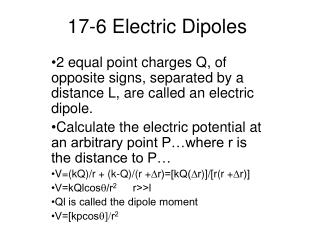# 17-6 Electric Dipoles - PowerPoint PPT PresentationDownload Presentation17-6 Electric Dipoles

Download Presentation## 17-6 Electric Dipoles

- - - - - - - - - - - - - - - - - - - - - - - - - - - E N D - - - - - - - - - - - - - - - - - - - - - - - - - - -
##### Presentation Transcript

1. 17-6 Electric Dipoles 2 equal point charges Q, of opposite signs, separated by a distance L, are called an electric dipole. Calculate the electric potential at an arbitrary point P…where r is the distance to P… V=(kQ)/r + (k-Q)/(r +Dr)=[kQ(Dr)]/[r(r +Dr)] V=kQlcosq/r2 r>>l Ql is called the dipole moment V=[kpcosq]/r2

2. Dipole cont’d • See example 17-6 p512

3. 17-7 Capacitance • A capacitor, sometimes called a condensor, is a device that can store an electric charge and consists of two ll plates separated by a space and connected to two conducting wires. • The symbol for a capacitor –ll- • Q=CV where C is capacitance and the SI unit for capacitance is the farad (F) • Capacitance, C, is constant for a capacitor. • C=eo[A/d] where A is area and d is distance between plates and eo=8.85 x10-12C2/N.m2

4. Capacitance cont’d • See 17-7 p514

5. 17-8 Dielectrics • In most capacitors, there is an insulating sheet called a dielectric in-between the plates. • The value for Keo can be found on the table on page 514 and will be in the possibly useful page information C=Keo[A/d] • See Conceptural Example 17-8 p516

6. 17-9 Storage of Electricity • A charged capacitor stores electricity…it is usually discharged by depressing one or both plates to send the charge across the space. • U= energy= ½ QV= ½ CV2= ½ [Q2/C] • See Example 17-9 page 517 • The quantity Ad is the volume between the plates where an electric field exists… • u=energy density=energy/volume=1/2 eoE2

7. 17-10 Cathode-ray tube • A CRT operates by thermonic emission. • The negative electrode or cathode and a positive electrode called an anode exist as two plates in an evacuated tube. • When the negative electrode is heated it gives off electrons, originally called cathode rays. (See pg. 519) • An oscilloscope is a device for representing an electric signal on a screen, using a CRT.

8. 17-11 The ECG and EKG • Each time the heart beats, a change in electrical potential can be detected using electrodes near it. The record of these changes are called an electrocardiogram… or EKG or ECG. • Infarcts, which are dead regions of the heart become evident. • See QRS group diagram page 520…(contraction of the ventricle and T recovery.